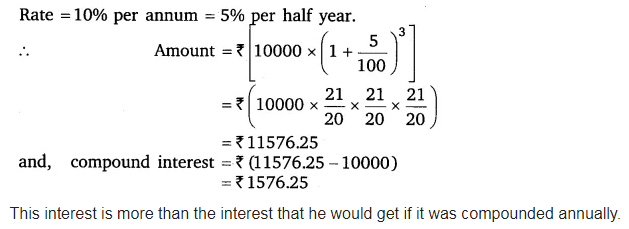# Find the amount and the compound interest on ₹10,000 for 11/2 years at 10% per annum, compounded half-yearly

Find the amount and the compound interest on ₹ 10,000 for 11/2 years at 10% per annum, compounded half-yearly. Would this interest be more than the interest he would get if it was compounded annually?

Here, Principal = ₹ 10000
Time = 1$\frac { 1 }{ 2 }$ years = 3 half years,